Practice the problems of Math in Focus Grade 1 Workbook Answer Key Chapter 14 Practice 1 Mental Addition to score better marks in the exam.

Then add the ones to the tens.

Example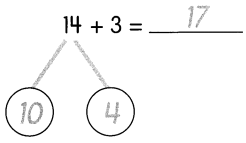Question 1.
15 + 2 = ____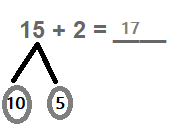Question 2.
12 + 4 = ____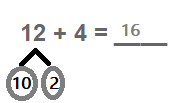Question 3.
35 + 1 = ___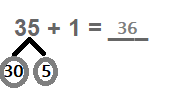Question 4.
23 + 5 = ____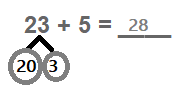Question 5.
22 + 7 = ___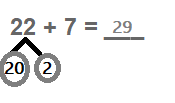Question 6.
31 + 8 = ___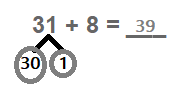Question 7.
6 + 32 = ____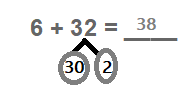Question 8.
5 + 34 = ____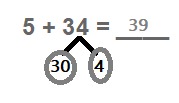Then add the tens to the ones.

Example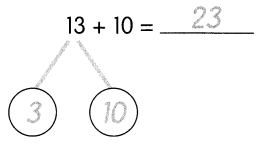Question 9.
18 + 10 = ____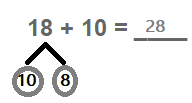Question 10.
11 + 20 = ____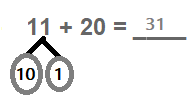Question 11.
12 + 10 = ____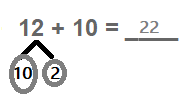Question 12.
14 + 20 = ____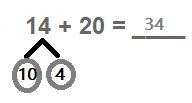Question 13.
16 + 10 = ____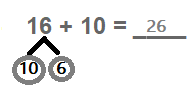Question 14.
20 + 19 = ____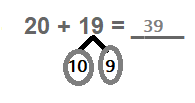Question 15.
10 + 17 = ___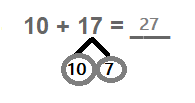Question 16.
20 + 13 = ____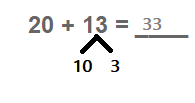Question 17.
10 + 14 = ____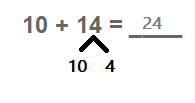Question 18.
20 + 16 = ____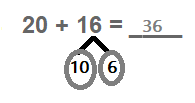Example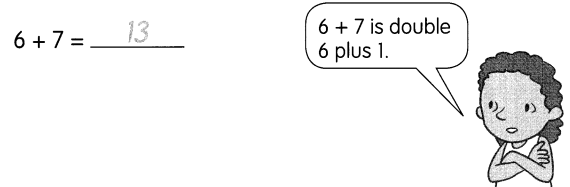Question 19.
4 + 5 = ____
By doubling and adding 4 with 4 we get 8,
Then adding 8 with 1 we get 9.

Question 20.
7 + 8 = _____
By doubling and adding 7 with 7 we get 14,
Then adding 14 with 1 we get 15.

Question 21.
5 + 6 = ___
By doubling and adding 5 with 5 we get 10,
Then adding 10 with 1 we get 11.

Question 22.
8 + 9 = ____
By doubling and adding 8 with 8 we get 16,
Then adding 16 with 1 we get 17.

Solve mentally. Fill in the blanks.

Question 23.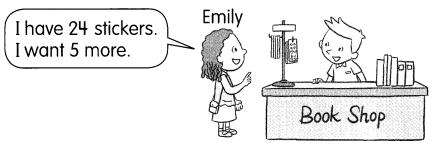How many stickers will Emily have? ____
Given,
Emily have 24 stickers and she needs 5 more,
By adding 24 with 5 we get 29,
Therefore, Emily will have 29 stickers in total.

Solve mentally. Fill in the blanks.

Question 24.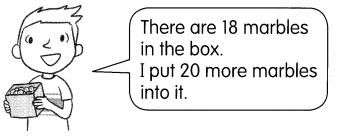How many marbles are there in the box now? ____
Given,
There are 18 marbles in the box,
And added 20 more marbles to it,
By adding 18 with 20 we get 38,
Therefore, there are 38 marbles in the box.

Question 25.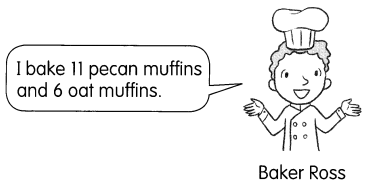How many muffins does Baker Ross bake in all? _____